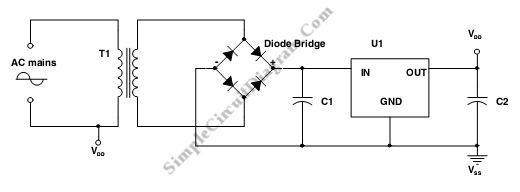# Linear Power Supply

A diode bridge, a step down transformer a linear regulator(U1) and some filtering capacitors can be used to build a Linear power supply. This circuit has some advantages such as this circuit has high output current and can give multiple output voltages easily. However it has some disadvantages like this is high standby losses, expensive circuit, and bulky size of 50 Hz transformer. Here is the  schematic diagram of the circuit:The output voltage(VDC), Transformer’s power(S) and power factor(pf) of a power supply determine the value of the output current. The power factor ussualy can be assumed 0.5 because of the high mains current harmmonic content. The output current is determined by following equation:
IDC=(Sxpf)/VDC

[Circuit’s schematic diagram source: STMicroelectronics Application Note]